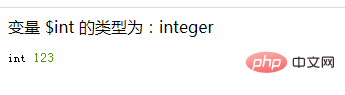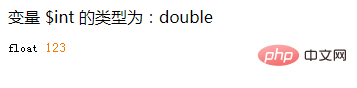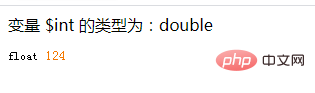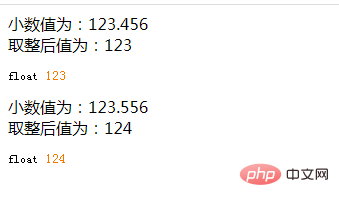# php怎么去掉小数位

php去掉小数位的方法：1、使用intval()函数获取变量的整数值部分，语法“intval(\$number)”；2、使用floor()函数向下取整，语法“floor(\$number)”。php去掉小数位的方法

intval() 函数用于获取变量的整数值。语法格式：

`int intval ( mixed \$var [, int \$base = 10 ] )`
• \$var：要转换成 integer 的数量值。

• \$base：转化所使用的进制。

如果 base 是 0，通过检测 var 的格式来决定使用的进制：

• 如果字符串包括了 "0x" (或 "0X") 的前缀，使用 16 进制 (hex)；否则，

• 如果字符串以 "0" 开始，使用 8 进制(octal)；否则，

• 将使用 10 进制 (decimal)。

intval()函数常被用来进行数据类型转换，将字符串、浮点数类型的变量转换为为整数类型。

```<?php
\$num = 123.456;
\$int = intval(\$num);
echo '变量 \$int 的类型为：'.gettype(\$int).'<br>';
var_dump(\$int);
?>```floor() 函数向下舍入为最接近的整数。语法格式：

`floor(\$number);`
• \$number ：必需。规定需向下取整的值。

```<?php
\$num = 123.456;
\$int = floor(\$num);
echo '变量 \$int 的类型为：'.gettype(\$int).'<br>';
var_dump(\$int);
?>```• 使用ceil()函数--向上取整，有小数就整数部分加1

```<?php
\$num = 123.456;
\$int = ceil(\$num);
echo '变量 \$int 的类型为：'.gettype(\$int).'<br>';
var_dump(\$int);
?>```• 使用round()函数--四舍五入

```<?php
\$num = 123.456;
\$int = round(\$num);
echo '小数值为：'.\$num.'<br>';
echo '取整后值为：'.\$int .'<br>';
var_dump(\$int);

\$num = 123.556;
\$int = round(\$num);
echo '小数值为：'.\$num.'<br>';
echo '取整后值为：'.\$int .'<br>';
var_dump(\$int);
?>```• 相关标签：php 去掉小数位 取整
• 相关文章

相关视频